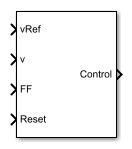# DC-DC Voltage Controller

Discrete-time DC-DC voltage PI control with feedforward and optional integral anti-windup

• Library:
• Simscape / Electrical / Control / General Machine Control

•## Description

The DC-DC Voltage Controller block implements discrete-time proportional-integral (PI) DC-DC voltage control with feedforward, FF. The feedforward input optimizes the transient response. The block can output a duty cycle or a current control signal. To avoid saturation of the integral gain, the block can implement anti-windup gain.### Equations

The equation that the DC-DC Voltage Controller block uses to calculate the control signal is

`$control=\left({K}_{p}+{K}_{i}\frac{{T}_{s}z}{z-1}\right)\left({v}_{ref}-v\right)+FF,$`

where:

• control is the control signal, expressed as a duty cycle or a current.

• Kp is the proportional gain.

• Ki is the integral gain.

• Ts is the sample time.

• vref is the reference voltage.

• v is the measured voltage.

• FF is the feedforward input.

To avoid saturation of the integrator output, the block uses an anti-windup mechanism. The integrator gain is then equal to

`${K}_{i}+{K}_{aw}\left(contro{l}_{sat}-contro{l}_{unsat}\right),$`

where:

• Kaw is the anti-windup gain.

• controlsat is the saturated control signal, which the block calculates as $contro{l}_{sat}=\text{min}\left(\mathrm{max}\left(contro{l}_{unsat},contro{l}_{min}\right),contro{l}_{max}\right),$

where:

• controlunsat is the unsaturated control signal.

• controlmin is the lower limit for the control signal.

• controlmax is the upper limit for the control signal.

## Ports

### Input

expand all

Desired DC output voltage for the plant.

Data Types: `single` | `double`

Measured DC output voltage for the plant.

Data Types: `single` | `double`

Feedforward term.

Data Types: `single` | `double`

External reset signal (rising edge) for the integrator.

Data Types: `single` | `double`

### Output

expand all

Control signal, control, expressed as a duty cycle or a current.

Data Types: `single` | `double`

## Parameters

expand all

Proportional gain, Kp, of the controller.

Integral gain, Ki, of the controller.

Anti-windup gain, Kaw, of the controller.

Upper limit for the Control output signal. The value must be greater than the value of the Control action lower limit parameter.

Lower limit for the Control output signal.

Time, in s, between consecutive block executions. During execution, the block produces outputs and, if appropriate, updates its internal state. For more information, see What Is Sample Time? and Specify Sample Time.

If this block is inside a triggered subsystem, inherit the sample time by setting this parameter to `-1`. If this block is in a continuous variable-step model, specify the sample time explicitly using a positive scalar.

Time constant, τ, for the DC voltage filter.

#### Dependencies

The Time constant voltage filter parameter is only visible when the Filter DC voltage checkbox is selected.

To enable the filter on voltage measurement path, select the checkbox. To disable the filter, clear the check box.

#### Dependencies

The Time constant voltage filter parameter is only visible when the Filter DC voltage check box is selected.

## Version History

Introduced in R2018a